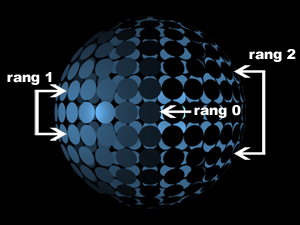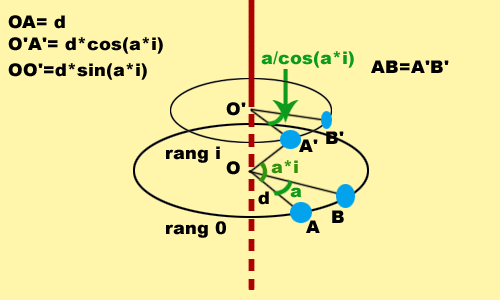Page 1 of 1

### Distribute layers in shape of sphere

Posted: February 10th, 2005, 10:38 am
This expression spreads layers in a 3D space to form a sphere. How does it do it? Simply by cutting a sphere in circular slices. These slices are, in my expression, the "rows". The "middle" slice is row 0, the slice above is row 1, and so forth.
As you can notice, apart from row 0, all the other rows are made of two slices.The sphere is symetrical to the place (x/z) containing row 0. My expression uses this symetry to facilitate the construction of the sphere.

Let's take a closer look :

The Null object "A" is in the middle of the sphere and is the parent of all the other layers. This allows to easily move and orient the sphere.

The value "a" allows to manage the space between two layers.

For the sphere to be perfectly homogeneous, this space has to be identical for all layers.
That is the first problem we encounter, because the radius of a slice depends on its row. Thus the spacing angle too.
The image below illustrates this problem : we want the AB distance to be equal to the A'B' distance.The radius of the "middle" slice (row 0) is d, the radius of the sphere.
But the radius of the i slice (row i) is d*cos(a*i).
So we obtain the following equation :

Code: Select all

``````d*a=d*cos(a*i)*x
``````
Where x is the spacing angle to row i.

So we get :

Code: Select all

``````x=a/cos(a*i)
``````
The second difficulty is the amount of layers per slice. The answer is simple, it is

Code: Select all

``````2*Math.PI/spacing angle
``````
For it to always be an integer, I added the Math.round function :

Code: Select all

``````s=Math.round(2*Math.PI/(a/Math.cos(a*i)))
``````
From there one has to adjust the spacing angle for each row, which becomes :

Code: Select all

``````u=2*Math.PI/s
``````
The symetry is obtained with the function Math.pow, Math.floor is the number of the layer : index-2 or n (n is the number of the layer, the first layer of the sphere is number 1...etc).
Here are the two lnes of code that create this symetry :

Code: Select all

``````symÃ©trie : y=u*Math.floor((index-2)/2)
``````
and

Code: Select all

``````d*Math.sin(a*rang)*Math.pow(-1,index-2)
``````
I hope this helped

Thanks

http://oscarvideo.free.fr/sphereoscarus.zip

Posted: February 16th, 2005, 9:43 am
I just had a chance to check out your project.

That is awesome!

Posted: February 16th, 2005, 11:24 am
thank you byronnash!

Oscarus.

### Congratulations !

Posted: May 17th, 2005, 8:41 am
This project give more power than the 3D layers assistants (in the case of a sphere of course) because you can animate all your layers all together..
Just amazing. Oscarus rocks and rules !

I give this script 5 smiles :UPDATE :
When moving the "angle"'s "curseur" to O or less, AE is freaking out, forcing me to quite the software.

### Re: Distribute layers in shape of sphere

Posted: October 1st, 2008, 2:23 am
Hi all!
great technique!
Quite dated thread, so I though to revamp it with my ignorance...

I downloaded the aep, and replaced the 47 blue layers with 47 other square ones, and pasted the expressions. After a take or two it worked without error warning, but the layers intersect, no matter how I adjust the sliders of the null.
the original layer size is 800x800 scaled to 576.
Is there any adjustment I need to do in order to get it right?
(using cs3)
Evan

### Re: Distribute layers in shape of sphere

Posted: May 31st, 2010, 11:40 am
Hello, I just realized a tutorial video with projet.aep showing how to create and animate a sphere with expression in After effects :sphere.flv

Oscarus
--------------------------------------------------------------------------
site: http://oscarvideo.free.fr
email: oscarvideo@free.fr
--------------------------------------------------------------------------

### Re: Distribute layers in shape of sphere

Posted: April 2nd, 2012, 10:33 am
oscarus wrote:Hello, I just realized a tutorial video with projet.aep showing how to create and animate a sphere with expression in After effects :sphere.flv

Oscarus
--------------------------------------------------------------------------
site: http://oscarvideo.free.fr
email: oscarvideo@free.fr
--------------------------------------------------------------------------
Looks really interesting, but I can't seem to find the English version. Any ideas?

John